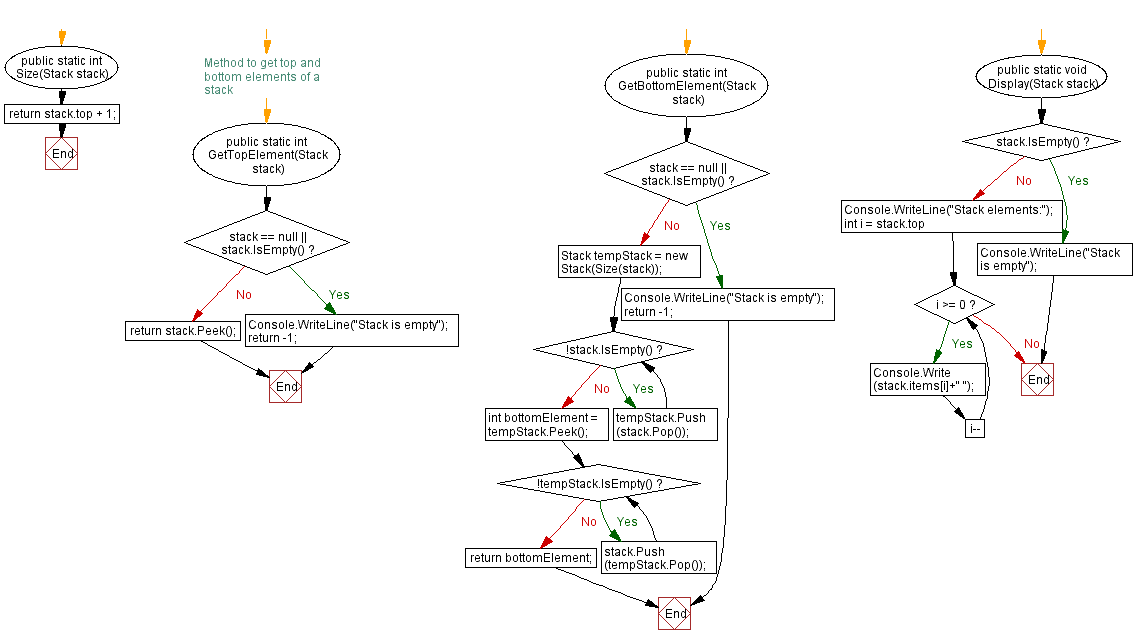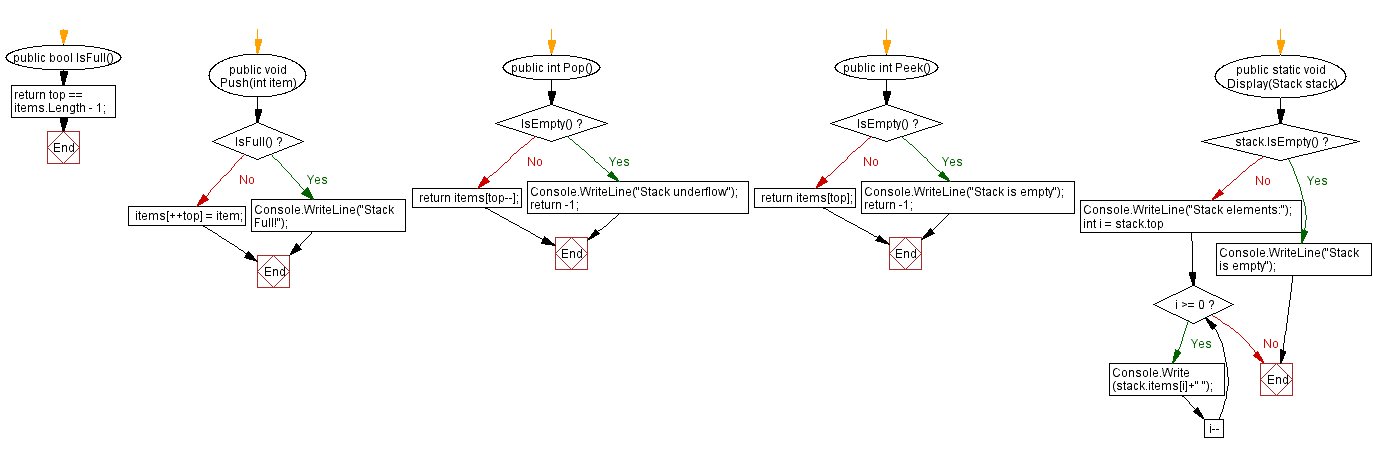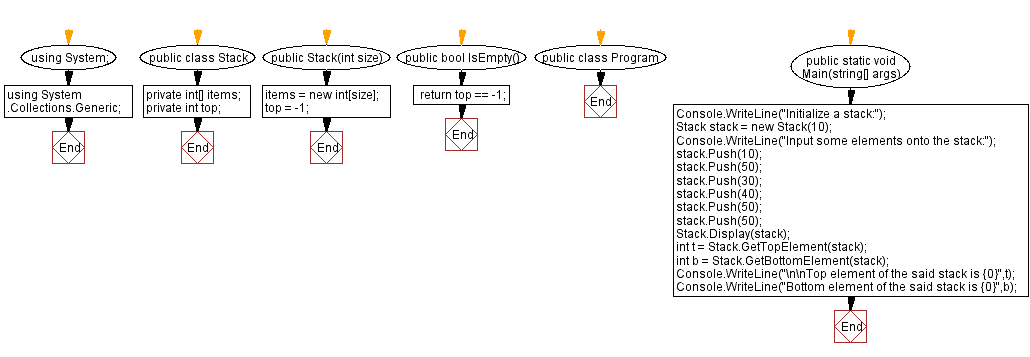﻿ C# - Top and bottom elements of a stack

# C# Sharp Stack Exercises: Top and bottom elements of a stack

## C# Sharp Stack: Exercise-13 with Solution

Write a C# program to find the top and bottom elements of a given stack.

Sample Solution:

C# Code:

``````using System;
using System.Collections.Generic;
public class Stack
{
private int[] items;
private int top;

public Stack(int size)
{
items = new int[size];
top = -1;
}

public bool IsEmpty()
{
}

public bool IsFull()
{
}

public void Push(int item)
{
if (IsFull())
{
Console.WriteLine("Stack Full!");
return;
}

items[++top] = item;
}

public int Pop()
{
if (IsEmpty())
{
Console.WriteLine("Stack underflow");
return -1;
}

return items[top--];
}

public int Peek()
{
if (IsEmpty())
{
Console.WriteLine("Stack is empty");
return -1;
}

return items[top];
}

public static int Size(Stack stack)
{
return stack.top + 1;
}

// Method to get top and bottom elements of a stack
public static int GetTopElement(Stack stack)
{
if (stack == null || stack.IsEmpty())
{
Console.WriteLine("Stack is empty");
return -1;
}

return stack.Peek();
}

public static int GetBottomElement(Stack stack)
{
if (stack == null || stack.IsEmpty())
{
Console.WriteLine("Stack is empty");
return -1;
}

Stack tempStack = new Stack(Size(stack));

while (!stack.IsEmpty())
{
tempStack.Push(stack.Pop());
}

int bottomElement = tempStack.Peek();

while (!tempStack.IsEmpty())
{
stack.Push(tempStack.Pop());
}

return bottomElement;
}

public static void Display(Stack stack)
{
if (stack.IsEmpty())
{
Console.WriteLine("Stack is empty");
return;
}

Console.WriteLine("Stack elements:");
for (int i = stack.top; i >= 0; i--)
{
Console.Write(stack.items[i]+" ");
}
}

}

public class Program
{
public static void Main(string[] args)
{
Console.WriteLine("Initialize a stack:");
Stack stack = new Stack(10);
Console.WriteLine("Input some elements onto the stack:");
stack.Push(10);
stack.Push(50);
stack.Push(30);
stack.Push(40);
stack.Push(50);
stack.Push(50);
Stack.Display(stack);
int t =  Stack.GetTopElement(stack);
int b = Stack.GetBottomElement(stack);
Console.WriteLine("\n\nTop element of the said stack is {0}",t);
Console.WriteLine("Bottom element of the said stack is {0}",b);
}
}
``````

Sample Output:

```Initialize a stack:
Input some elements onto the stack:
Stack elements:
50 50 40 30 50 10

Top element of the said stack is 50
Bottom element of the said stack is 10
```

Flowchart:C# Sharp Code Editor:

Improve this sample solution and post your code through Disqus

What is the difficulty level of this exercise?

Test your Programming skills with w3resource's quiz.

﻿